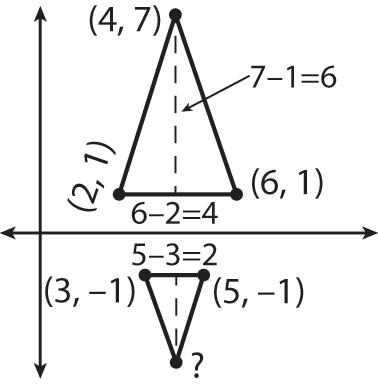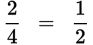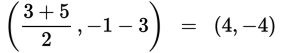# SAT Math Multiple Choice Question 968: Answer and Explanation

### Test Information

Question: 968

4. Triangle CAT is an isosceles triangle with vertices (2, 1), (6, 1), and (4, 7). Triangle DOG is similar to triangle CAT, and two of its vertices are (3, –1) and (5, –1). If the third vertex has a y-coordinate that is less than –1, what are the coordinates of the third vertex?

• A. (3, -4)
• B. (3, -5)
• C. (4, -4)
• D. (4, -5)

Explanation:

C

Difficulty: Hard

Category: Additional Topics in Math / Geometry

Strategic Advice: Drawing a sketch is the only way to approach a question like this.

Getting to the Answer: Even though you don't have graph paper, draw a quick sketch to get an idea of how the triangles look. Don't forget that the question tells you that the y-coordinate of the third vertex is less than -1, so you know the third vertex is below the other two vertices. Also, label any distances that are obvious from the coordinates of the points. Your sketch might look like the following:Now, examine the figure more closely. The question states that the triangles are similar, so look for the ratio of the corresponding sides. The ratio of the base of the smaller triangle to the base of the larger triangle is, so the ratio of the other pairs of sides, and therefore the height, must be the same. This means the height of triangle DOG is half the height of triangle CAT, or half of 6, which is 3 (which means the vertex is 3 units below -1). The vertex that creates the height is exactly halfway between the two base vertices, so the coordinates of the third vertex is, which is (C).